0
1897

# Geometry Questions for XAT

Download important Geometry Questions for XAT PDF based on previously asked questions in the XAT exam. Practice Geometry Questions  PDF for the XAT exam.

Question 1: Three horses are grazing within a semi-circular field. In the diagram given below, AB is the diameter of the semi-circular field with center at O. Horses are tied up at P, R and S such that PO and RO are the radii of semi-circles with centers at P and R respectively, and S is the center of the circle touching the two semi-circles with diameters AO and OB. The horses tied at P and R can graze within the respective semi-circles and the horse tied at S can graze within the circle centred at S. The percentage of the area of the semi-circle with diameter AB that cannot be grazed by the horses is nearest to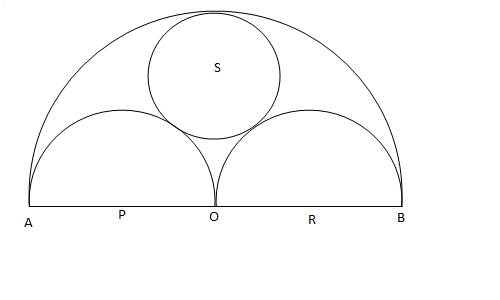a) 20

b) 28

c) 36

d) 40

Question 2: In the figure below, AB is the chord of a circle with center O. AB is extended to C such that BC = OB. The straight line CO is produced to meet the circle at D. If $\angle{ACD}$ = y degrees and $\angle{AOD}$ = x degrees such that x = ky, then the value of k is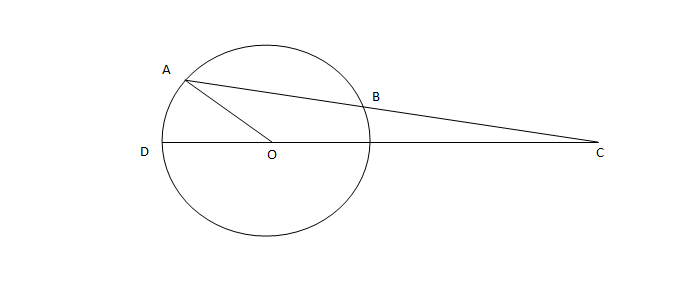a) 3

b) 2

c) 1

d) None of the above.

Question 3: If the lengths of diagonals DF, AG and CE of the cube shown in the adjoining figure are equal to the three sides of a triangle, then the radius of the circle circumscribing that triangle will be?a) equal to the side of the cube

b) $\sqrt 3$ times the side of the cube

c) 1/$\sqrt 3$ times the side of the cube

d) impossible to find from the given information

Question 4: A circle with radius 2 is placed against a right angle. Another smaller circle is also placed as shown in the adjoining figure. What is the radius of the smaller circle?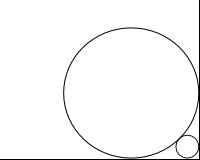a) $3 – 2 \sqrt{2}$

b) $4 – 2 \sqrt{2}$

c) $7- 4 \sqrt{2}$

d) $6- 4 \sqrt{2}$

Question 5: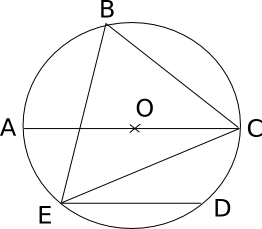In the adjoining figure, chord ED is parallel to the diameter AC of the circle. If angle CBE = 65°, then what is the value of angle DEC?

a) 35

b) 55

c) 45

d) 25

Question 6: The figure shows a circle of diameter AB and radius 6.5 cm. If chord CA is 5 cm long, find the area of triangle ABC.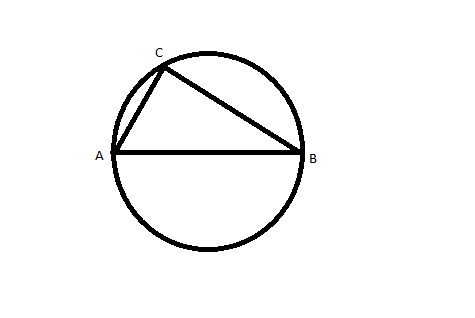a) 60 sq. cm

b) 30 sq. cm

c) 40 sq. cm

d) 52 sq. cm

Question 7: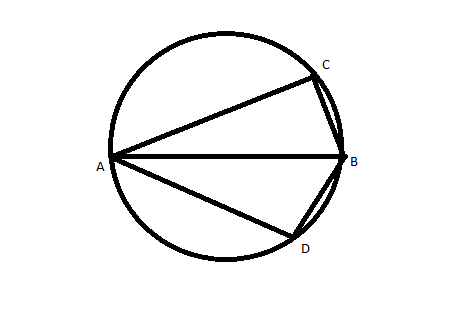AB is the diameter of the given circle, while points C and D lie on the circumference as shown. If AB is 15 cm, AC is 12 cm and BD is 9 cm, find the area of the quadrilateral ACBD.

a) 54sq. cm

b) 216sq. cm

c) 162sq. cm

d) None of these

Question 8: In the adjoining figure, points A, B, C and D lie on the circle. AD = 24 and BC = 12. What is the ratio of the area of CBE to that of ADE?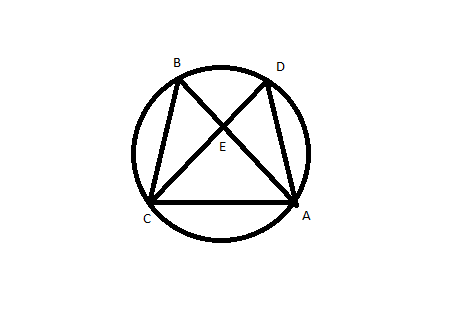a) 1 : 4

b) 1 : 2

c) 1 : 3

d) Data insufficient

Question 9: Three circles, each of radius 20, have centres at P, Q and R. Further, AB = 5, CD = 10 and EF = 12. What is the perimeter of ΔPQR ?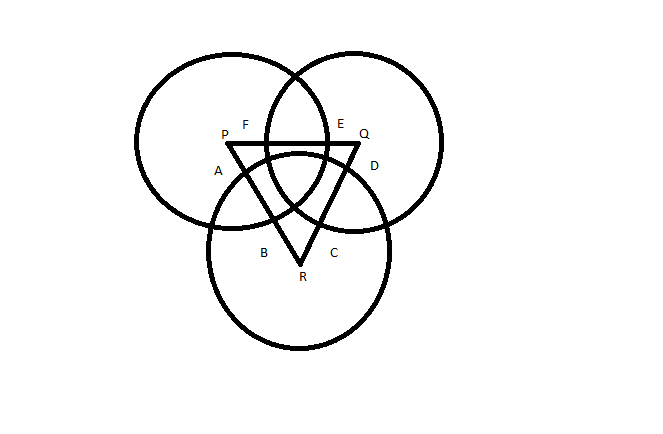a) 120

b) 66

c) 93

d) 87

Question 10:

In a circular field, AOB and COD are two mutually perpendicular diameters having length of 4 meters. X is the mid – point of OA. Y is the point on the circumference such that ∠YOD = 30°. Which of the following correctly gives the relation among the three alternate paths from X to Y?

a) XOBY : XODY : XADY :: 5.15 : 4.50 : 5.06

b) XADY : XODY : XOBY :: 6.25 : 5.34 : 4.24

c) XODY : XOBY : XADY :: 4.04 : 5.35 : 5.25

d) XADY : XOBY : XODY :: 5.19 : 5.09 : 4.04

e) XOBY : XADY : XODY :: 5.06 : 5.15 : 4.50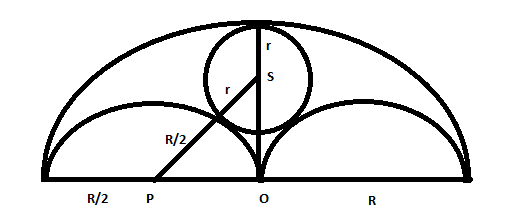Let R be radius of big circle and r be radius of circle with centre S. Radius of 2 semicircles is R/2.

From Right angled triangle OPS, using pythagoras theorem we get

$(r+0.5R)^2 = (0.5R)^2 + (R-r)^2$ . We get R=3r .

Now the area of big semicircle that cannot be grazed is Area of big S.C – area of 2 semicircle – area of small circle = $\pi*R^2$/2 – 2*$\pi*(0.5R)^2$/2-$\pi*r^2$ = $\pi*R^2$/2 – 2*$\pi*(0.5R)^2$/2-$\pi*(R/3)^2$= $\pi*R^2$/2 – $\pi*(R)^2$/4-$\pi*(R)^2$/9 = 5*$\pi*R^2$/36.  this is about 28 % of the area $\pi*R^2$/2 . Hence option B.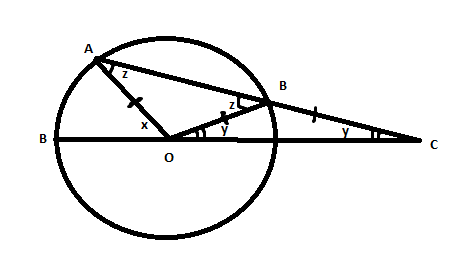Since Angle BOC = Angle BCO = y.

Angle OBC = 180-2y .

Hence Angle ABO =  z = 2y = Angle OAB.

Now since x is exterior angle of triangle AOC .

We have x = z + y = 3y.

Hence option A.

Consider side of the cube as x.

So diagonal will be of length $\sqrt{3}$ * x.

Now if diagonals are side of equilateral triangle we get area = 3*$\sqrt{3}*x^2$ /4 .

Also in a triangle

4 * Area * R = Product of sides

4* 3*$\sqrt{3}*x^2$ /4 * R = .3*$\sqrt{3}*x^3$

R = x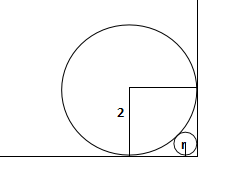Using pythagoras we can find diagonal of small square shown in fig. = 2*$\sqrt2$ . Now this is equal to 2 + r +  $\sqrt2$ *r. Equating we get r = $6- 4 \sqrt{2}$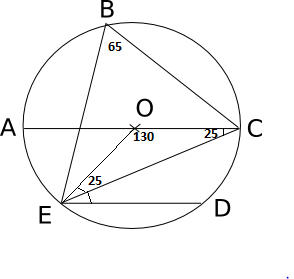If EBC = 65 then EOC = 130 then OEC = OCE = 25. NOw since OC and ED are parallel we have OCE = OED = 25. Hence option D.

According to given dimensions, triangle will be a right angled triangle.
So BC = 12
And area = $\frac{1}{2} \times 12 \times 5$
= 30

Since ACBD is cyclic quadrilateral with diagonals as AB = 15 ad CD.
So area = $\frac{1}{2} \times (AB) \times (CD)$                  eq.(1)
Triangle ACB and ABD is right angled triangle
Let’s say angle CBA = $\theta$
so $CD = 2 \times (9 sin\theta)$  (Where  $sin\theta$ = $\frac{12}{15}$)
CD = $\frac{72}{5}$
Putting values in eq. (1) , we will get area = 108 sq.cm.

As we know angles of same sectors are equal
Hence angle B and angle D will be equal. Angle BCE and angle EAD will be equal.
So triangles BCE and EAD will be similar triangles with sides ratio as 12:24 or 1:2.
Area will be in ratio of 1:4.